# Formulas Tab in Microsoft Excel

We use Formula tab to insert functions, define the name, create the name range, review the formula, etc. In ribbon, Formulas tab has very important and most useful functions to make dynamic reports.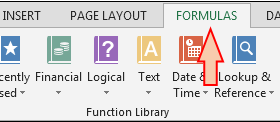Under the formulas tab we have 4 groups:-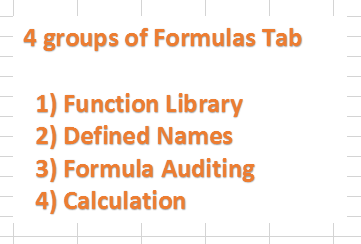a)   Function Library: -In Excel, we have 461 functions and they are available in the formulas tab under the function library group. And there is no need to learn every formula for we can find formulas category wise such as:- Financial, Logical, Text, Date & Time, Lookup & Reference, Math & Trig, many more.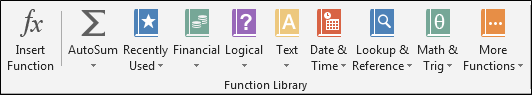b)   Defined Names: -  We use this option to define the name of a cell, range and the list of the already defined name can be viewed in Name manager and we can edit it if we want to change the range or cell reference of any defined name. Also, we can use the defined name in Formulas.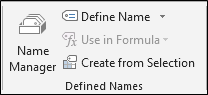c)   Formula Auditing: - This option helps us to identify the relation of formulas. By using Trace precedent, we get to know on which cell formula cell is based. Trace dependents are used to know if active cell is being used in any formula. When we use trace precedent and trace dependents the arrows get inserted automatically and, to remove the arrows, we use Remove Arrows. Show formula is a self-explained word; it is used to show the formula in the sheet. Error checking is used to check the error in the sheet. Evaluate formula option is used to evaluate the formula step by step.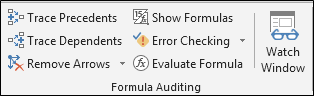d)   Calculation: -If we want to switch the calculation from automatically to manually calculation and manually to automatically calculation, then we can do it by using the Calculation option. We use Calculate Now and Calculate Sheet option if automatic calculation is turned off.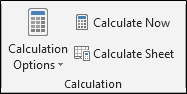We would love to hear from you, do let us know how we can improve, complement or innovate our work and make it better for you. Write us at info@exceltip.com

## Users are saying about us...

1.awesome

2.for the life of me, I CANNOT find the defined names box on the formulas ribbon of excel 365, so I can't change the name of the range. Where is it???????

Terms and Conditions of use

The applications/code on this site are distributed as is and without warranties or liability. In no event shall the owner of the copyrights, or the authors of the applications/code be liable for any loss of profit, any problems or any damage resulting from the use or evaluation of the applications/code.